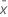# Methods and formulas for 1-Sample t

Select the method or formula of your choice.

## Confidence interval (CI)

### Notation

TermDescriptionsample mean
t α/2 inverse cumulative probability of a t distribution with n-1 degrees of freedom at 1-α/2
α 1 – confidence level/100
s sample standard deviation
n sample size

## T-value

### Notation

TermDescriptionsample mean
μ 0 hypothesized population mean
s sample standard deviation
n sample size

## P-value

### Formula

The calculation for the p-value depends on the alternative hypothesis.

Alternative Hypothesis P-value

### Notation

TermDescription
μ population mean
μ0 hypothesized population mean
tt-statistic of the sample data
tn-1a random variable from the t-distribution with n-1 degrees of freedom
nsample size
By using this site you agree to the use of cookies for analytics and personalized content.  Read our policy